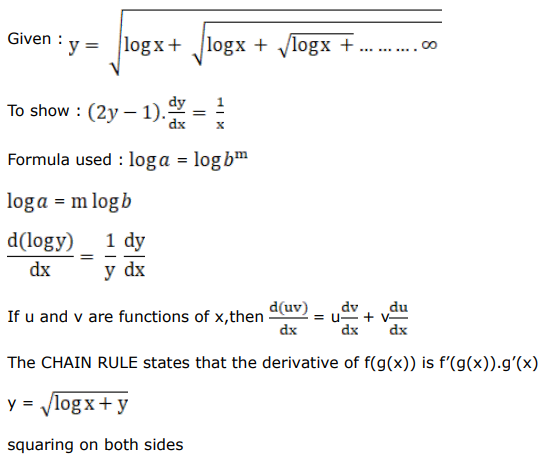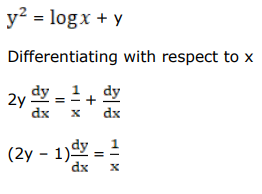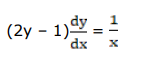# Solve this followingQuestion:

If $y=\sqrt{\log x+\sqrt{\log x+\sqrt{\log x+\ldots \infty}}}$, show that $(2 y-1) \cdot \frac{d y}{d x}=\frac{1}{x}$.

Solution: